Solving Complicated Proportions
 Home > Lessons > Solving Complicated Proportions Search | Updated December 22nd, 2018
Introduction

In this section of MATHguide, you will learn about proportions. Here are the topics within this page:

 Proportions Proportions are used for a variety of goals: determining a 'normal' weight for a baby at a certain age, calculating distances on maps, identifying inventory rates for businesses, ...     MATHguide has a lessons that explains the mathematical nature of proportions, if you require a refresher on the topic.esson: Proportions     The sections that follow will allow you to understand how to solve complicated proportions that lead to linear and quadratic equations. Solving Complicated Proportions Leading to Linear Equations The second type of complicated proportion leads to linear equations. Before progressing, you will need to know how to solve linear equations. Review this lesson, if necessary, before progressing.esson: Solving Linear Equations     Take a look at this example to see how to solve a complicated proportions. Example: Solve this proportion.We know from our knowledge of proportions (see Proportions) that cross-products must be equal. So, cross-multiply.Cross-multiplying results with these two equal products.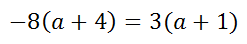The steps that follow will indicate how the linear equation can be solved for the a-value. Do the distributive property.Subtract 3a from both sides of the equation.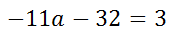Add 32 to both sides of the equation.Divide both sides of the equation by -11.Rounded to the nearest 10th, the solution is...This video will inform you as to how to solve this type of complicated proportion.ideo: Solving Complicated Proportions     Try these interactive quizmasters to determine if you have the hang of it.uiz: Proportions 2uiz: Proportions 2 with Help Solving Complicated Proportions Leading to Quadratic Equations The first type of complicated proportion leads to quadratic equations. Before progressing, you will need to know how to solve quadratic equations. Review this lesson, if necessary, before progressing.esson: Solving Quadratic Equations     Review this example, which will demonstrate how to solve complicated proportions. Example: Solve this proportion.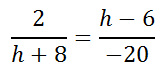Like the problem in the previous section (see Solving Complicated Proportions), we can use the cross-products to begin on our journey to solve for the h-value.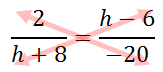Here are those cross-products.Multiply the two binomials. A multiplication table is best here (see Multiplying Polynomials for help).Add 40 to both sides of the equation.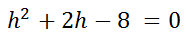Now, factor the trinomial (see Factoring Trinomials for help).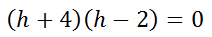Next, we set the factors equal to zero (see for help).Last, we solve each equation separately to gain two solutions.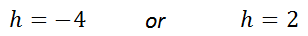Note, be careful to look at the denominators of our original problem. We have to be careful not to get a zero in a denominator because zero denominators are illogical and therefore unacceptable.     If we substitute h=-4 into the original problem, the denominator is not equal to zero, making it a possible solution. For the same reason, h=2 is another acceptable solution.     This video will inform you as to how to solve this type of complicated proportion.ideo: Solving Complicated Proportions 2     Try this interactive quizmaster to determine if you digested the lesson above.uiz: Proportions 3 Instructional Videos Here are instructional videos that explain all the aspects of solving complicated proportions.ideo: Solving Complicated Proportionsideo: Solving Complicated Proportions 2 Interactive Quizmasters Try these quizmasters to determine if you understand how to solve complicated proportions.uiz: Proportions 2uiz: Proportions 2 with Helpuiz: Proportions 3 Related Lessons These lessons are related to solving complicated proportions.esson: Areas and Sides of Similar Figuresesson: Proportionsesson: Similar Figuresesson: Dilationsesson: Solving Linear Equationsesson: Factoring Quadratic Polynomialsesson: Multiplying Binomialsesson: Solving Quadratic Equationsesson: Areas and Lengths of Similar Figuresesson: Volumes and Lengths of Similar Solids